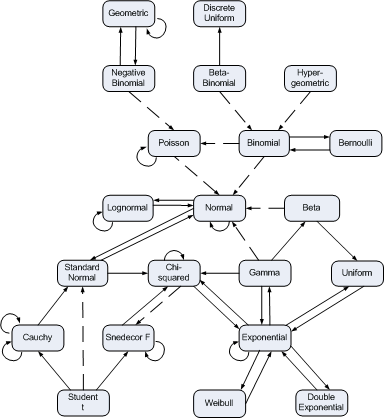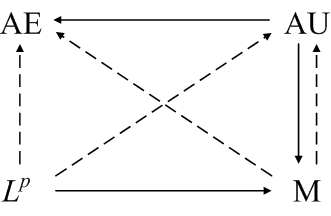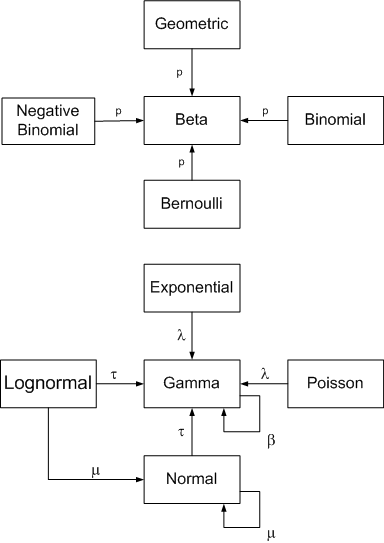# Three math diagrams

Here are three pages containing diagrams that each summarize several theorems.

The distribution relationships page summarizing around 40 relationships between around 20 statistical distributions.The modes of convergence page has three diagrams like the following that explain when one kind of convergence implies another: almost everywhere, almost uniform, Lp, and convergence in measure.The page conjugate prior relationships is similar to the probability distribution page, but concentrates on relationships in Bayesian statistics.What are some of your favorite diagrams? Do you have any suggestions for diagrams that you’d like to see someone make?